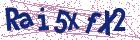# Singularly perturbed two-point boundary value problem by applying exponential fitted finite difference method

Document Type : Research Article

Authors

1 Department of Mathematics, National Institute of Technology Patna, Patna - 800005, India.

2 Department of Science and Technology, Bihar, Government Polytechnic, Lakhisarai, Lakhisarai- 811311, India.

Abstract

The present study addresses an exponentially fitted finite difference method to obtain the solution of singularly perturbed two-point boundary value problems (BVPs) having a boundary layer at one end (left or right) point on uniform mesh. A fitting factor is introduced in the derived scheme using the theory of singular perturbations. Thomas algorithm is employed to solve the resulting tri-diagonal system of equations. The convergence of the presented method is investigated. Several model example problems are solved using the proposed method. The results are presented with terms of maximum absolute errors, which demonstrate the accuracy and efficiency of the method. It is observed that the proposed method is capable of producing highly accurate results with minimal computational effort for a fixed value of step size h, when the perturbation parameter tends to zero. From the graphs, we also observed that a numerical solution approximates the exact solution very well in the boundary layers for smaller value of ε.

Keywords

Main Subjects

#### References

 Andargie, A. and Reddy, Y. Two initial value problems approach for solving singular perturbations problems, Am. J. Comput. Math. 2(03) (2012), 213–216.
 Bender, C.M. and Orsazag, S.A. Advanced mathematical methods for scientists and engineers, Springer, New York, 1999.
 Chakravarthy, P. and Reddy, Y.N. Exponentially fitted modified upwind scheme for singular perturbation problems, Int. J. Fluid Mech. Res. 33 (2006), 119–136.
 Gold, R.R. Magneto hydrodynamic pipe flow, Part I. J. Fluid Mech. 13 (1962), 505–512.
 Habib, H.M. and El-Zahar, E.R. An algorithm for solving singular perturbation problems with mechanization, Appl. Math. Comput. 188 (2007), 286–302.
 Hinch, E.J. Perturbation methods, Cambridge University Press, Cam-bridge, 1991.
 Holmes, M.H. Introduction to perturbation methods, Springer, Berlin, 1995.
 Jayakumar, J. and Ramanujam, N. A numerical method for singular perturbation problems arising in chemical reactor theory, Comput. Math. Appl. 27 (1994,) 83–99.
 Kadalbajoo, M.K. and Kumar, D. Initial value technique for singularly perturbed two point boundary value problems using an exponentially fitted finite difference scheme, Comput. Math. Appl. 57 (2009), 1147–1156.
 Kadalbajoo, M.K. and Kumar, D. A brief survey on numerical meth-ods for solving singularly perturbed problems, Appl. Math. Comput. 217 (2010), 3641–3716.
 Kevorkian, J. and Cole, J.D. Perturbation methods in applied mathemat-ics, 2nd Edition, Springer-Verlag, New York, 1981.
 Kumar, M. and Surabhi, T. An initial-value technique to solve third-order reaction-diffusion singularly perturbed boundary-value problems, Int. J. Comput. Math. 89(17) (2012), 2345–2352.
 Kumar, M., Singh, P. and Hradyesh Kumar, M. An initial-value tech-nique for singularly perturbed boundary value problems via cubic spline, Int. J. Comput. Methods Eng. Sci. Mech. 8(6) (2007), 419–427.
 Lorenz, J. Combinations of initial and boundary value methods for a class of singular perturbation problems, Numerical analysis of singular pertur-bation problems (Proc. Conf., Math. Inst., Catholic Univ., Nijmegen, 1978), pp. 295–315, Academic Press, London-New York, 1979.
 Madhu Latha, K., Phaneendra, K. and Reddy, Y.N. Numerical inte-gration with exponential fitting factor for singularly perturbed two point boundary value problems, British Journal of Mathematics & Computer Science 3(3) (2013), 397–414.
 Miller, J.J.H. Singular perturbation problems in chemical physics, ana-lytic and computational methods, XCVII Wiley, New York, 1997.
 Miller, J.J.H., Riordan, R.E.O. and Shishkin, G.I. Fitted numerical methods for singular perturbation problems, error estimates in the max-imum norm for linear problems in one and two dimensions, World Sci-entific, 1996.
 Mishra, H. and Saini, S. Numerical solution of singularly perturbed two-point boundary value problem via Liouville-Green transform, Am. J. Comput. Math. 3(1) (2013), 1–5.
 Nayfeh, A.H. Perturbation methods, John Wiley & Sons, Inc., New York, 1979.
 Nayfeh, A.H. Introduction to perturbation techniques, Wiley-VCH, New York, 1993.
 O’Malley, R.E. Introduction to singular perturbations, Academic Press, New York, 1974.
 O’Malley, R.E. Singular perturbation methods for ordinary differential equations, Applied Mathematical Sciences, 89, Springer, Berlin, 1990.
 Padmaja, P., Aparna, P. and Gorla, R.S.R. An initial-value technique for self-adjoint singularly perturbed two-point boundary value problems, Int. J. Appl. Mech. Eng. 25(1) (2020), 106–126.
 Phaneendra, K. and Lalu, M. Gaussian quadrature for two-point singu-larly perturbed boundary value problems with exponential fitting, Com-munications in Mathematics and Applications 10(3) (2019), 447–467.
 Ranjan, R. and Prasad, H.S. An efficient method of numerical integration for a class of singularly perturbed two point boundary value problems, WSEAS Trans. Math. 17 (2018), 265–273.
 Ranjan, R. and Prasad, H.S., A fitted finite difference scheme for solving singularly perturbed two point boundary value problems, Inf. Sci. Lett. 9(2), (2020), 65–73.
 Ranjan, R., Prasad, H.S. and Alam, J. A simple method of numerical integration for a class of singularly perturbed two point boundary value problems, i-manager’s Journal on Mathematics 7(1) (2018), 41.
 Roos, H.G., Stynes, M. and Tobiska, L. Numerical methods for singularly perturbed differential equations, Springer, Berlin 1996.
 Smith, D.R. Singular perturbation theory: An introduction with applica-tions, Cambridge University Press, Cambridge, 1985.
 Verhulst, F. Methods and applications of singular perturbations: Bound-ary layers and multiple timescale dynamics, Springer, Berlin 2005.
 Vigo-Aguiar, J. and Natesan, S. An efficient numerical method for singu-lar perturbation problems, J. Comput. Appl. Math., 192 (2006), 132–141.